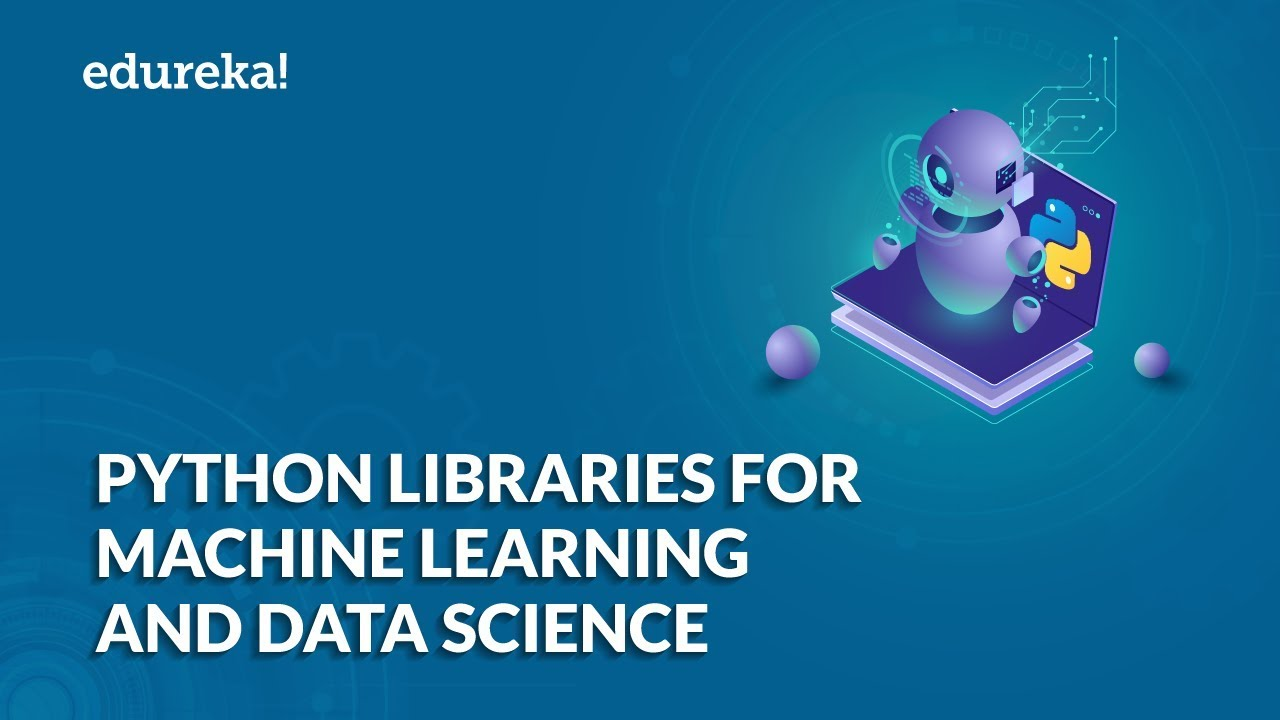# Mathematics for Machine Learning - Essential Math for Machine LearningMathematics for Machine Learning - Essential Math for Machine Learning: teaches you all the math needed to get started with mastering Machine Learning. It teaches you all the necessary topics and concepts of Linear Algebra, Multivariate Calculus, Statistics, and Probability and also dives into the actual implementation of these topics.

This Edureka video on 'Mathematics for Machine Learning' teaches you all the math needed to get started with mastering Machine Learning. It teaches you all the necessary topics and concepts of Linear Algebra, Multivariate Calculus, Statistics, and Probability and also dives into the actual implementation of these topics.

## Machine Learning, Data Science and Deep Learning with PythonComplete hands-on Machine Learning tutorial with Data Science, Tensorflow, Artificial Intelligence, and Neural Networks. Introducing Tensorflow, Using Tensorflow, Introducing Keras, Using Keras, Convolutional Neural Networks (CNNs), Recurrent Neural Networks (RNNs), Learning Deep Learning, Machine Learning with Neural Networks, Deep Learning Tutorial with Python

Machine Learning, Data Science and Deep Learning with Python

### Complete hands-on Machine Learning tutorial with Data Science, Tensorflow, Artificial Intelligence, and Neural Networks

Explore the full course on Udemy (special discount included in the link): http://learnstartup.net/p/BkS5nEmZg

In less than 3 hours, you can understand the theory behind modern artificial intelligence, and apply it with several hands-on examples. This is machine learning on steroids! Find out why everyone’s so excited about it and how it really works – and what modern AI can and cannot really do.

In this course, we will cover:
• Deep Learning Pre-requistes (gradient descent, autodiff, softmax)
• The History of Artificial Neural Networks
• Deep Learning in the Tensorflow Playground
• Deep Learning Details
• Introducing Tensorflow
• Using Tensorflow
• Introducing Keras
• Using Keras to Predict Political Parties
• Convolutional Neural Networks (CNNs)
• Using CNNs for Handwriting Recognition
• Recurrent Neural Networks (RNNs)
• Using a RNN for Sentiment Analysis
• The Ethics of Deep Learning
• Learning More about Deep Learning

At the end, you will have a final challenge to create your own deep learning / machine learning system to predict whether real mammogram results are benign or malignant, using your own artificial neural network you have learned to code from scratch with Python.

Separate the reality of modern AI from the hype – by learning about deep learning, well, deeply. You will need some familiarity with Python and linear algebra to follow along, but if you have that experience, you will find that neural networks are not as complicated as they sound. And how they actually work is quite elegant!

This is hands-on tutorial with real code you can download, study, and run yourself.

## Python For Data Science - How to use Data Science with PythonThis Edureka video on 'Python For Data Science - How to use Data Science with Python - Data Science using Python ' will help you understand how we can use python for data science along with various use cases. What is Data Science? Why Python? Python Libraries For Data Science. Roadmap To Data Science With Python. Data Science Jobs and Salary Trends

This Edureka video on 'Python For Data Science - How to use Data Science with Python - Data Science using Python
' will help you understand how we can use python for data science along with various use cases. Following are the topics discussed this Python Data Science Tutorial:

• What is Data Science?
• Why Python?
• Python Libraries For Data Science
• Roadmap To Data Science With Python
• Data Science Jobs and Salary Trends
• How Edureka Helps?

## Best Python Libraries For Data Science & Machine LearningBest Python Libraries For Data Science & Machine Learning | Data Science Python Libraries

This video will focus on the top Python libraries that you should know to master Data Science and Machine Learning. Here’s a list of topics that are covered in this session:

• Introduction To Data Science And Machine Learning
• Why Use Python For Data Science And Machine Learning?
• Python Libraries for Data Science And Machine Learning
• Python libraries for Statistics
• Python libraries for Visualization
• Python libraries for Machine Learning
• Python libraries for Deep Learning
• Python libraries for Natural Language Processing